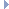# OpenGraph DrawingFramework

v. 2022.02 (Dogwood)

ogdf::steiner_tree::SaveEnum< T > Class Template Reference

This class computes save edges recursively and stores for every node pair their save edge in a HashArray. More...

#include <ogdf/graphalg/steiner_tree/SaveEnum.h>Inheritance diagram for ogdf::steiner_tree::SaveEnum< T >:

## Public Member Functions

SaveEnum (EdgeWeightedGraphCopy< T > &steinerTree)
Initializes the data structures and calculates a MST of the given complete terminal graph. More...

virtual ~SaveEnum ()

virtual T gain (node u, node v, node w) const
Returns the gain (sum of the save edges) of a node triple by an table lookup. More...

void rebuild ()
Rebuild the lookup table (necessary if the tree has changed) More...

virtual edge saveEdge (node u, node v) const
Determines the save edge between two nodes by a table lookup. More...

virtual T saveWeight (node u, node v) const
Determines the weight of the save edge between two nodes by a table lookup. More...

virtual void update (const Triple< T > &t)
Updates the weighted tree data structure given a contracted triple. More...Public Member Functions inherited from ogdf::steiner_tree::Save< T >
Save ()

virtual ~Save ()

## Protected Member Functions

void build ()
Build the lookup table. More...

void buildRecursively (EdgeArray< bool > &hidden, node u, List< node > &processedNodes)
Builds the lookup table for the save edges recursively. More...

## Private Attributes

Array2D< edgem_save
Data structure for the lookup table. More...

EdgeWeightedGraphCopy< T > * m_steinerTree
The current terminal spanning tree. More...

## Detailed Description

### template<typename T> class ogdf::steiner_tree::SaveEnum< T >

This class computes save edges recursively and stores for every node pair their save edge in a HashArray.

Definition at line 48 of file SaveEnum.h.

## ◆ SaveEnum()

template<typename T >
 ogdf::steiner_tree::SaveEnum< T >::SaveEnum ( EdgeWeightedGraphCopy< T > & steinerTree )
inlineexplicit

Initializes the data structures and calculates a MST of the given complete terminal graph.

Parameters
 steinerTree the given terminal spanning tree

Definition at line 55 of file SaveEnum.h.

## ◆ ~SaveEnum()

template<typename T >
 virtual ogdf::steiner_tree::SaveEnum< T >::~SaveEnum ( )
inlinevirtual

Definition at line 63 of file SaveEnum.h.

## ◆ build()

template<typename T >
 void ogdf::steiner_tree::SaveEnum< T >::build ( )
inlineprotected

Build the lookup table.

Definition at line 145 of file SaveEnum.h.

## ◆ buildRecursively()

template<typename T >
 void ogdf::steiner_tree::SaveEnum< T >::buildRecursively ( EdgeArray< bool > & hidden, node u, List< node > & processedNodes )
inlineprotected

Builds the lookup table for the save edges recursively.

This is done by first finding the maximum weighted edge in the graph. This edge partitions the graph and is the save edge for each pair of nodes such that not both of the nodes come from the same partition. This procedure is then applied to both partitions recursively.

Parameters
 hidden Bool array of hidden edges u Starting node for traversing a partition in order to find a maximum weighted edge processedNodes List of seen nodes during the traversing (all nodes of the component)

Definition at line 165 of file SaveEnum.h.

## ◆ gain()

template<typename T >
 virtual T ogdf::steiner_tree::SaveEnum< T >::gain ( node u, node v, node w ) const
inlinevirtual

Returns the gain (sum of the save edges) of a node triple by an table lookup.

Parameters
 u First triple node v Second triple node w Third triple node
Returns
Sum of the save edges between the three nodes

Implements ogdf::steiner_tree::Save< T >.

Definition at line 100 of file SaveEnum.h.

## ◆ rebuild()

template<typename T >
 void ogdf::steiner_tree::SaveEnum< T >::rebuild ( )
inline

Rebuild the lookup table (necessary if the tree has changed)

Definition at line 117 of file SaveEnum.h.

## ◆ saveEdge()

template<typename T >
 virtual edge ogdf::steiner_tree::SaveEnum< T >::saveEdge ( node u, node v ) const
inlinevirtual

Determines the save edge between two nodes by a table lookup.

Parameters
 u First terminal v Second terminal
Returns
The save edge between two given nodes

Implements ogdf::steiner_tree::Save< T >.

Definition at line 86 of file SaveEnum.h.

## ◆ saveWeight()

template<typename T >
 virtual T ogdf::steiner_tree::SaveEnum< T >::saveWeight ( node u, node v ) const
inlinevirtual

Determines the weight of the save edge between two nodes by a table lookup.

Parameters
 u First terminal v Second terminal
Returns
Weight of the save edge between two given nodes

Implements ogdf::steiner_tree::Save< T >.

Definition at line 73 of file SaveEnum.h.

## ◆ update()

template<typename T >
 virtual void ogdf::steiner_tree::SaveEnum< T >::update ( const Triple< T > & t )
inlinevirtual

Updates the weighted tree data structure given a contracted triple.

The update first inserts two 0-cost edges into the complete terminal graph and removes the two save edges. Afterward the lookup table is rebuild.

Parameters
 t The contracted triple

Implements ogdf::steiner_tree::Save< T >.

Definition at line 130 of file SaveEnum.h.

## ◆ m_save

template<typename T >
 Array2D ogdf::steiner_tree::SaveEnum< T >::m_save
private

Data structure for the lookup table.

Definition at line 215 of file SaveEnum.h.

## ◆ m_steinerTree

template<typename T >
 EdgeWeightedGraphCopy* ogdf::steiner_tree::SaveEnum< T >::m_steinerTree
private

The current terminal spanning tree.

Definition at line 216 of file SaveEnum.h.

The documentation for this class was generated from the following file: